Next: THE CENTRAL-LIMIT THEOREM Up: Resolution Previous: FREQUENCY-STATISTICAL RESOLUTION

# TIME-FREQUENCY-STATISTICAL RESOLUTION

Many time functions are not completely random from point to point but become more random when viewed over a longer time scale. A popular mathematical model embodying this concept is to make a so-called stationary time series by putting random numbers into a filter as depicted in Figure 5. The input xt may be independent random numbers or white light. [The two terms mean nearly the same thing in practice but the first term is the stronger; it means that xt is in no way related to xs if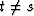, whereas white light means that E(xt xs) = 0 if.] The output random time series yt may vary rather slowly from point to point if ft is a low-pass filter. This is the usual case when we are modeling continuous time functions. The random time series may be called a stationary random time series if neither the filter nor any property of the random numbers (such as m or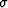) vary with time. Stationarity is often assumed even wwhere it cannot be strictly true.

This model will be useful later when we consider the problem of predicting a future point on yt from knowledge of past values. Now we will use the model to examine the estimation of the spectrum of yt given a sample of n points of yt. To begin with, we have a very precise meaning for the spectrum of yt. We have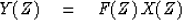(49)
and its conjugate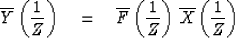(50)
Multiplying (49) by (50) we get(51)
but, from the previous section, we learned that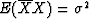.Considering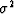to be unity, we see that the expected power spectrum of the output Y is the energy spectrum of the filter F. The overall situation is depicted in Figure 6. The interesting question is how well can we estimate the spectrum when we start with an n-point sample of yt. We will describe three computationally different methods, all having the same fundamental limitations.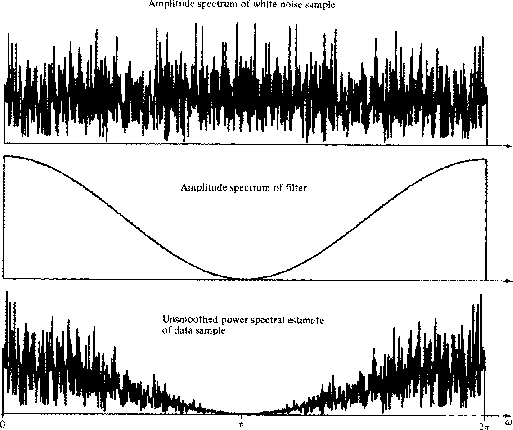4-5
Figure 6
Spectral estimation.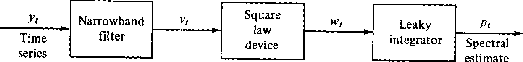4-6
Figure 7
Spectral estimate of a random series.The first method uses a bank of filters as shown in Figure 7. When random numbers excite the narrowband filter, the output is somewhat like a sine wave. It differs in one important respect. A sine wave has constant amplitude, but the output of a narrowband filter has an amplitude which swings over a range. This is illustrated in Figure 8. If the bandwidth is narrow, the amplitude changes slowly. If the impulse response of the filter has duration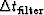,then the output amplitude at time t will be randomly related to the amplitude at time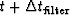.Thus, in statistical averaging, it is not the number of time points but the number of intervalswhich enhance the reliability of the average. Consequently, the decay time of the integrator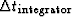will generally be chosen to be greater than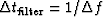.The variability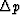of the output p decreases asincreases. Since vt has independent values over time spans of about,then the degrees of freedom'' smoothed over can be written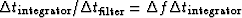.The variability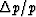is proportional to the inverse square root of the number of degrees of freedom, and so we get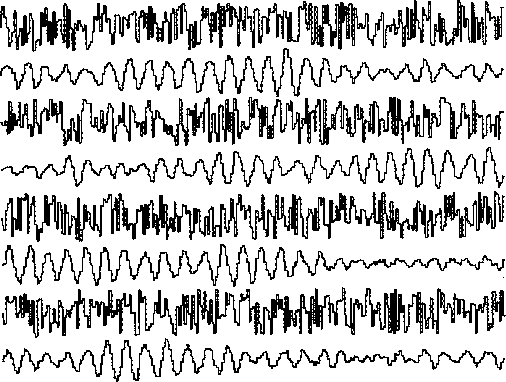4-7
Figure 8
1024 random numbers before and after narrowband filtering. The filter was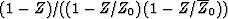where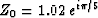.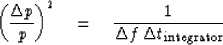or, introducing the usual inequality,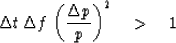(52)
The inequality (52) indicates the three-parameter uncertainty which is fundamental to estimating power spectra of random functions. Two other methods of estimating the spectrum of yt from a sample of length n are exactly the same as the methods described in Sec. 4-3 as ways of estimating the spectrum of white light. In fact, (52) turns out to be the same as (48).

The usual interpretation is that to attain a frequency resolution of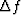and a relative accuracy ofa time sample of duration at least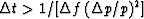will be required. Although this sort of interpretation is generally correct, it will break down for highly resonant series recorded for a short time. Then the data sample may be predictable an appreciable distance off its ends so that the effective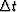is somewhat (perhaps appreciably) larger than the sample length.

## EXERCISES:

1. It is popular to taper the ends of a data sample so that the data go smoothly to zero at the ends of the sample. Choose a weighting function and discuss in a semiquantitative fashion its effect on,,and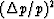.
2. Answer the question of Exercise 1, where the autocorrelation function is tapered rather than the data sample.Next: THE CENTRAL-LIMIT THEOREM Up: Resolution Previous: FREQUENCY-STATISTICAL RESOLUTION
Stanford Exploration Project
10/30/1997